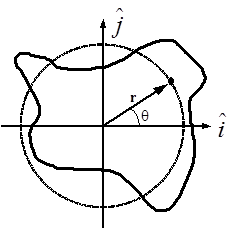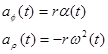$$\require{cancel}$$

# 01. Concepts and Principles

•• Contributed by Paul D'Alessandris
• Professor (Engineering Science and Physics) at Monroe Community College

# Rotation about a Fixed Axis (Spinning)

Imagine a rigid body constrained to rotate about a fixed axis. Non-physicists would say that the object is spinning.Place the origin of a coordinate system at the location of the rotation axis. Examine an arbitrary point on the object, denoted by the position vectororNotice that as the object spins, this point undergoes circular motion (denoted by the dashed line). Although the actual object may be of irregular shape, as it spins every point on the object undergoes circular motion. Moreover, since each and every point on the object has to complete an entire cycle around the rotation axis in the same amound of time, every point must undergo circular motion with the same angular speed (w(t)) and the same angular acceleraton (a(t)).

Since every point on a rigid body must have the same angular speed and the same angular acceleration, we will speak of the angular speed and angular acceleration of the object, rather than the angular speed and angular acceleration of some point on the object.

Given that every point on a spinning object undergoes circular motion, the results from our study of circular motion will be very important in analyzing spinning objects. Recall that with q(t) defined as the angular position of an arbitrary point on the rigid-body,andMoreover, the velocity and acceleration of any point on a spinning, rigid body can be related to the angular quantities:and# Rotation and Translation

To describe the motion of an object undergoing pure rotation (spinning), we have to describe the circular motion each point on the object undergoes. What must we do if the object is simultaneously rotating and translating (moving in a plane perpendicular to the rotation axis), like a wheel rolling down an incline?

The answer lies in the independence of these two types of motion. In much the same manner as we attacked kinematics in two dimensions by independently analyzing the horizontal and vertical motions, we will attack rigid-body kinematics by independently analyzing the rotational and translational motions. In short, we will model any motion of a rigid-body as a superposition of a translation of the object's center-of-mass (CM) (which we will analyze by particle kinematics) and a rotation about an axis passing through the CM (which we will analyze by the kinematics of spinning, detailed above).For example, examine the motion of the thin rod between t1 and t2. Although the rod may have been spinning crazily through space between these two times, we can model its motion as a superposition of a simple translation of its CM without rotation (denoted by the vector Dr), which leaves the rod in the orientation denoted by the dashed lines, and a simple rotation about an axis through its CM without translation (denoted by Dq), which leaves the rod in its proper, final orientation. If we imagine the time difference (t2 - t1) shrinking toward zero, hopefully it becomes plausible that we can model any motion through this method.

In summary, to describe the motion of an arbitrary rigid body we will break the motion down into a pure translation of the CM and a pure rotation about the CM. We will use particle kinematics to describe the rotational portion. The velocity (or acceleration) of any point on the object is then determined by the sum of the velocity ( or acceleration) due to the translation and the velocity ( or acceleration) due to the rotation.